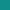## All numbers are created equalLet x = 1

Now multiply both sides by x;

x2 = x

Substract 1 from both sides;

x2 – 1 = x – 1

Use the identity x2 – 1 = (x + 1)(x – 1);

(x + 1)(x – 1) = (x – 1)

Cancel the factors (x – 1);

(x + 1) = 1

But x was equal to 1; so

2 = 1

q. e. d.## Objections